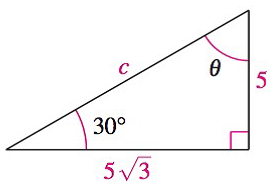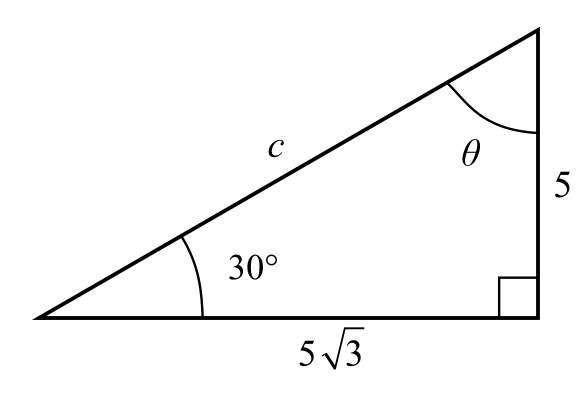Chapter 8.1, Problem 33E### Calculus: An Applied Approach (Min...

10th Edition
Ron Larson
ISBN: 9781305860919

#### Solutions

Chapter
Section### Calculus: An Applied Approach (Min...

10th Edition
Ron Larson
ISBN: 9781305860919
Textbook Problem
1 views

# Analyzing Triangles In Exercises 33-40, solve the triangle for the indicated side and/or angle.To determine

To calculate: The values of side c and angle θ in a triangle provided below.Explanation

Given Information:

The provided triangle is shown below,

Formula used:

In a right triangle, the sum of two acute angles is 90°.

Pythagorean theorem for any right triangle is such that c2=a2+b2, where a, b are the two perpendicular sides of a triangle and c is the length of hypotenuse.

Calculation:

Consider the sides of triangle, 53, 5 and c

The value of side a is 53, side b is 5 and hypotenuse is c.

Substitute a=53 and b=5 in the equation c2=a2+b2

### Still sussing out bartleby?

Check out a sample textbook solution.

See a sample solution

#### The Solution to Your Study Problems

Bartleby provides explanations to thousands of textbook problems written by our experts, many with advanced degrees!

Get Started

#### Prove the identity. 48. tan2 sin2 = tan2 sin2

Single Variable Calculus: Early Transcendentals, Volume I

#### In Exercises 63-68, rationalize the denominator. 68. 2xy

Applied Calculus for the Managerial, Life, and Social Sciences: A Brief Approach

#### The graph of x = cos t, y = sin2 t is:

Study Guide for Stewart's Single Variable Calculus: Early Transcendentals, 8th